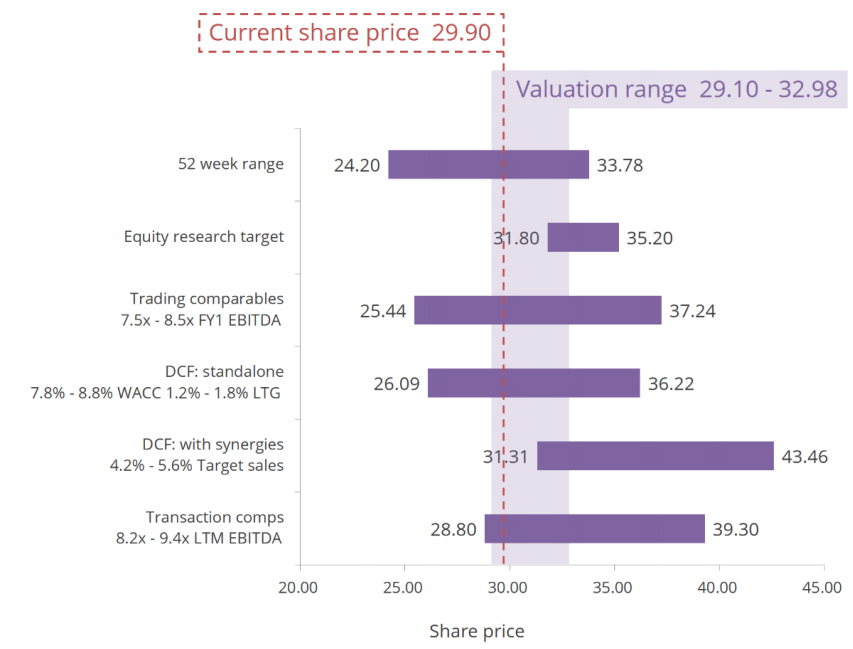## What is a Football Field?

A football field is a graph used to compare and summarize the results of different valuation methodologies to value a company. It is prepared using a floating bar chart in Excel and summarizes a range of values for a company, based on different valuation methodologies and assumptions. The football field is commonly used in investment banking. The underlying purpose of the chart is to give a visual representation of the valuation range, average, and target valuation for the company/asset.

Examples of valuation methodologies included in a football field are trading comparables, transaction comparables, 52-week trading ranges, and discounted cash flow (DCF) among others. The estimates and forecasts related to the valuation process of a company are subject to imprecision, uncertainties, and errors, which necessitate the use of more than one valuation methodology.

## Key Learning Points

• A football field is a graphical representation of the results from various valuation techniques.
• A football field not only shows the valuation range but also states the mean valuation arrived at using different valuation methodologies.
• A football field should include the range of valuation methodologies used, assumptions made, the current share price of the company, and highlight the acquisition value range.

## Why is a Football Field Important?

The valuation process involves making a significant number of forecasts and estimates to measure the financial performance of a company. Analysts make estimates about a firm’s business performance, the macroeconomic environment, market demand, and many other factors. However, these estimates are prone to uncertainties and are ultimately dependent on the analyst. Future cash flows could vary from estimates for various reasons including lower-than-expected demand (resulting in decreased sales), technological disruption, regulatory changes, etc.

To deal with such uncertainties and reduce the margin for error, analysts may use more than one valuation methodology, such as those stated above, for valuing a single company.

Next, different methodologies have their own assumptions and estimates. For example, the DCF method could involve different estimates of the terminal growth rate, resulting in multiple estimates of terminal value (which is the estimated value of a company beyond the forecast period) and the trading comparables methodology applies a low to high range of multiples.

Having stated the above, the use of different valuation methods and assumptions results in a wide valuation range. A football field shows the range and the mean valuation arrived at using different methodologies (and their underlying assumptions) in a single chart. This process helps in reducing the margin for error and also assists the decision-maker in choosing the most appropriate method to achieve the best valuation.

## Football Field – Example

Given below is an example of a valuation football field:A football field should include the following:

### Valuation Techniques

The left-hand side (vertical axis) or the y-axis lists the different valuation methods and assumptions used by an analyst to value a company. In the example given above, the analyst has used six different valuation methods.

Using the trading comparables method, the FY1 EBITDA multiples range from 7.5x to 8.5x. The Discounted Cash Flow (DCF) standalone method estimates the Weighted Average Cost of Capital (WACC) of between 7.8% to 8.8% and applies long-term growth (LTG) estimates of between 1.2% and 1.8%. The DCF with the synergies method applies projected sales growth of between 4.2% and 5.6%. The transaction comparables method applies an LTM EBITDA range of 8.2x to 9.4x.

### Units

The x-axis (horizontal axis) features the unit used for measuring value. For public companies, the unit used is the share price, as in the above example. For private companies, the unit used is enterprise value (EV.) The current share price in this football field is shown as a vertical floating line and is mentioned clearly (i.e. 29.90 in this example) to aid in decision-making. Further, one can gather from the football field that some of the valuation methods stated above indicate an implied value that is below the current share price.

### Acquisition Valuation Range

Finally, the football field should highlight the acquisition value range. In the aforesaid example, the valuation range is between 29.10 and 32.98 per share. Notably, this range overlaps with the results obtained from all the valuation methodologies mentioned above.